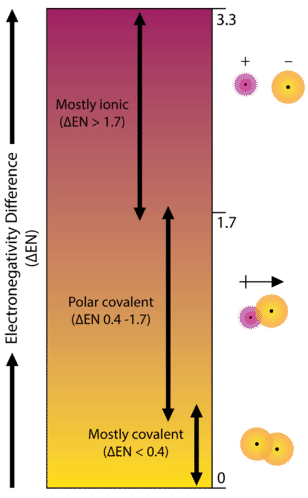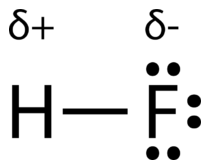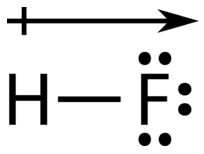# 9.5 Bond Polarity

$$\newcommand{\vecs}{\overset { \rightharpoonup} {\mathbf{#1}} }$$ $$\newcommand{\vecd}{\overset{-\!-\!\rightharpoonup}{\vphantom{a}\smash {#1}}}$$$$\newcommand{\id}{\mathrm{id}}$$ $$\newcommand{\Span}{\mathrm{span}}$$ $$\newcommand{\kernel}{\mathrm{null}\,}$$ $$\newcommand{\range}{\mathrm{range}\,}$$ $$\newcommand{\RealPart}{\mathrm{Re}}$$ $$\newcommand{\ImaginaryPart}{\mathrm{Im}}$$ $$\newcommand{\Argument}{\mathrm{Arg}}$$ $$\newcommand{\norm}{\| #1 \|}$$ $$\newcommand{\inner}{\langle #1, #2 \rangle}$$ $$\newcommand{\Span}{\mathrm{span}}$$ $$\newcommand{\id}{\mathrm{id}}$$ $$\newcommand{\Span}{\mathrm{span}}$$ $$\newcommand{\kernel}{\mathrm{null}\,}$$ $$\newcommand{\range}{\mathrm{range}\,}$$ $$\newcommand{\RealPart}{\mathrm{Re}}$$ $$\newcommand{\ImaginaryPart}{\mathrm{Im}}$$ $$\newcommand{\Argument}{\mathrm{Arg}}$$ $$\newcommand{\norm}{\| #1 \|}$$ $$\newcommand{\inner}{\langle #1, #2 \rangle}$$ $$\newcommand{\Span}{\mathrm{span}}$$

Have you ever spent time with someone you really didn't like? You had nothing in common with them and did not want to have anything to do with them. On the other hand, there are people you enjoy being with. You have a lot in common and like to share with them. Atoms work the same way. If there are strong differences in their attraction of electrons, on atom gets the electrons and the other atom loses them. If they are similar, they share electrons to form a covalent bond.

## Bond Polarity

Electronegativity is defined as the ability of an atom to attract electrons when the atoms are in a compound. Electronegativities of elements are shown in the periodic table below.Figure 9.13.1: Electronegativities of elements.

The degree to which a given bond is ionic or covalent is determined by calculating the difference in electronegativity between the two atoms involved in the bond.

As an example, consider the bond that occurs between an atom of potassium and an atom of fluorine. Using the table, the difference in electronegativity is equal to $$4.0 - 0.8 = 3.2$$. Since the difference in electronegativity is relatively large, the bond between the two atoms is ionic. Since the fluorine atom has a much larger attraction for electrons than the potassium atom does, the valence electron from the potassium atom is completely transferred to the fluorine atom. The diagram below shows how difference in electronegativity relates to the ionic or covalent character of a chemical bond.Figure 9.13.2: Bond type is predicted on the difference in electronegativity of the two elements involved in the bond.

The polarity of a covalent bond can be judged by determining the difference of the electronegativities of the two atoms involved in the covalent bond, as summarized in the following table:

Electronegativity Difference Bond Type
0 nonpolar covalent
0–0.4 slightly polar covalent
0.4–1.9 definitely polar covalent
>1.9 likely ionic

### Nonpolar Covalent Bonds

A bond in which the electronegativity difference is less than 1.7 is considered to be mostly covalent in character. However, at this point we need to distinguish between two general types of covalent bonds. A nonpolar covalent bond is a covalent bond in which the bonding electrons are shared equally between the two atoms. In a nonpolar covalent bond, the distribution of electrical charge is balanced between the two atoms.Figure 9.13.3: A nonpolar covalent bond is one in which the distribution of electron density between the two atoms is equal.

The two chlorine atoms share the pair of electrons in the single covalent bond equally, and the electron density surrounding the $$\ce{Cl_2}$$ molecule is symmetrical. Also note that molecules in which the electronegativity difference is very small (<0.4) are also considered nonpolar covalent. An example would be a bond between chlorine and bromine ($$\Delta$$EN $$=3.0 - 2.8 = 0.2$$).

### Polar Covalent Bonds

A bond in which the electronegativity difference between the atoms is between 0.4 and 1.7 is called a polar covalent bond. A polar covalent bond is a covalent bond in which the atoms have an unequal attraction for electrons and so the sharing is unequal. In a polar covalent bond, sometimes simply called a polar bond, the distribution of electrons around the molecule is no longer symmetrical.Figure 9.13.4: In the polar covalent bond of $$\ce{HF}$$, the electron density is unevenly distributed. There is a higher density (red) near the fluorine atom, and a lower density (blue) near the hydrogen atom.

An easy way to illustrate the uneven electron distribution in a polar covalent bond is to use the Greek letter delta $$\left( \delta \right)$$.Figure 9.13.5: Use of $$\delta$$ to indicate partial charge.

The atom with the greater electronegativity acquires a partial negative charge, while the atom with the lesser electronegativity acquires a partial positive charge. The delta symbol is used to indicate that the quantity of charge is less than one. A crossed arrow can also be used to indicate the direction of greater electron density.Figure 9.13.6: Use of crossed arrow to indicate polarity.

## Summary

• The electronegativity of an atom determines how strongly it attracts electrons to itself.
• The polarity of a bond is affected by the electronegativity values of the two atoms involved in that bond.

## Contributors

9.5 Bond Polarity is shared under a CC BY-NC license and was authored, remixed, and/or curated by LibreTexts.